# Turbulence

## Introduction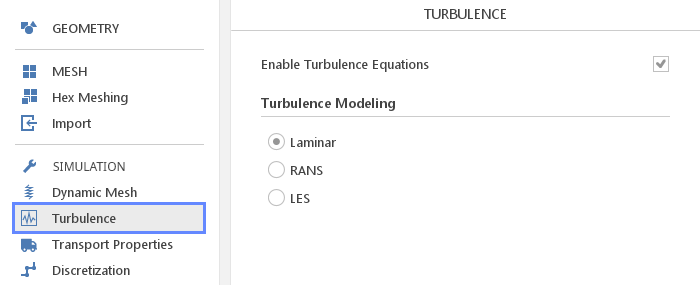In the Turbulence panel you can enable turbulence models appropriate for your simulation.

## Enabling and Disabling Turbulence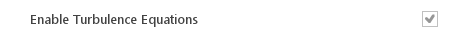In certain situations it can be useful to disable turbulence model at the beginning of simulation. This can be done using the Enable Turbulence Equations button and is usually used to stabilize simulation. Turbulence model can be enabled or disabled even while the simulation is still running.

## Turbulence Modeling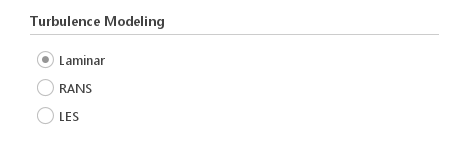You can select one of three approaches to turbulence modelling:

• Laminar - no turbulence modeling is used, applicable for very low velocity flows

• RANS - Reynolds-Averaged Navier-Stokes is most widely used modeling approach, which offers balance between accuracy and computational cost

• LES - Large Eddy Simulation offers improved modelling of large turbulent structures but is very computationally intensive

## RANS Models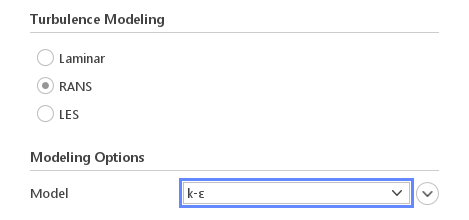When you select RANS turbulence modeling you will only have to define a Model .

Currently available models are:

• $$k-\epsilon$$

• $$RNG k-\epsilon$$

• $$Realizable k-\epsilon$$

• $$Spalart-Allmaras$$

• $$k-\omega$$

• $$k-\omega SST$$

• $$k-\omega SST SAS$$

• $$k-kl-\omega$$

Available models will vary depending on the version of OpenFOAM you are using and the solver that is currently selected.

### Model Constants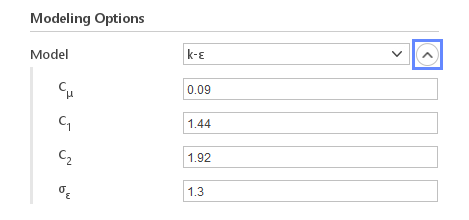Most models require some constants to be specified. You can edit these constants by clicking the Expand button. Usually, you should not change the default values.

## LES Models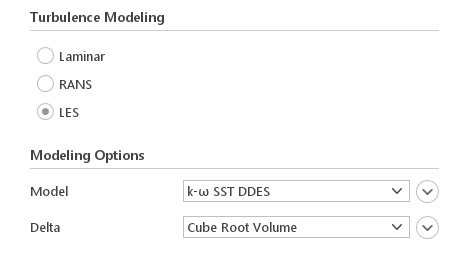When you select LES modeling you will be able to specify:

• Model - LES or DES model to use

• Delta - method for determining cutoff length scale

• Filter – if selected model requires, you can select type of the LES filter

Currently, available LES models:

• $$k-\omega SST DDES$$

• $$k-\omega SST IDDES$$

• $$k-\omega SST DES$$

• $$Spalart-Allmaras DES$$

• $$Spalart-Allmaras DDES$$

• $$Spalart-Allmaras IDDES$$

• $$Smagorinsky$$

• $$WALE$$

• $$k Equation$$

• $$Dynamic k Equation$$

### Delta Calculation MethodsCurrently, available Delta calculation methods:

• Cube Root Volume

• Max Delta XYZ

Inputs:

• Coefficient

### Filter Operators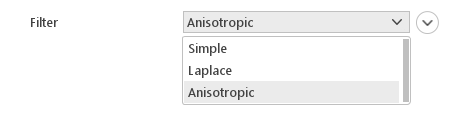For Dynamic k Equation model there are available Filter operators:

• Simple

• Laplace

• Anisotropic

Inputs:

• Width Coefficient